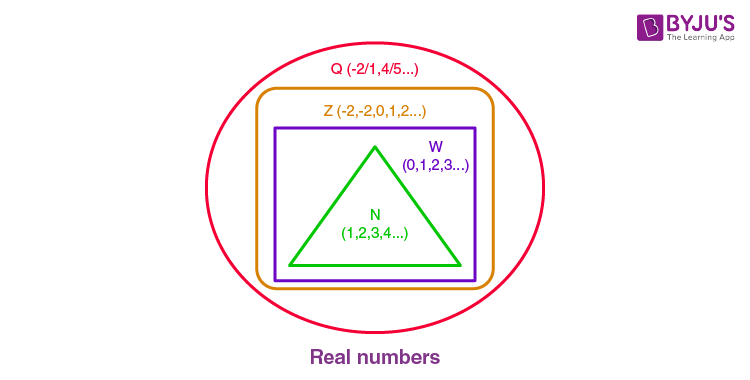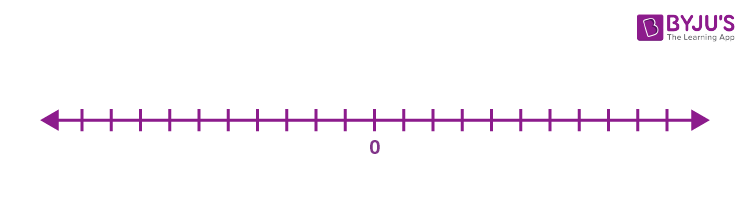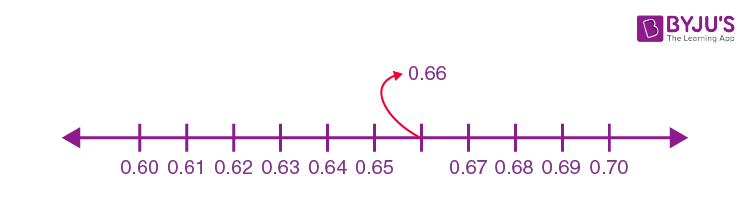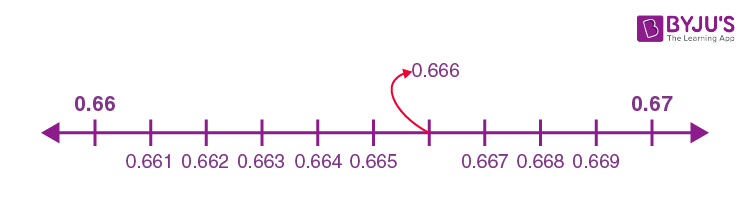# Rational Numbers on a Number Line

Rational Numbers on a Number Line: A rational number is any real number that can be expressed as a simple fraction or ratio. By definition, a rational number is a number which can be written in the form of p/q, where p and q are any two integers, and q is not equal to zero (q ≠ 0). Rational numbers can be positive or negative. If we take a closer look at counting numbers, all of them are rational numbers with denominator 1. The set of rational numbers is denoted by the letter “Q”.## Representation of Rational Numbers on a Number Line

Like the representation of the whole numbers, integers on the number line, the rational numbers can also be represented on the number line. In the number line, the number zero (0) is called the origin. All the positive values are represented on the right side of the origin, and the negative ones are on the left side. Now, let us learn how to represent the rational numbers on the number line.

The representation of rational numbers can be classified into two different types. The rational number may be in a proper fraction or improper fraction.

If it is a proper fraction, the numerator value is less than the denominator value; hence, the given rational number should be less than 1 and greater than zero. We can easily represent the given rational number on the number line.

If it is an improper fraction, the numerator value is greater than the denominator value; hence, the given rational number should be greater than 1. So, in this case, convert the improper fraction into a mixed one. This conversion helps to locate the exact position of the given fraction on the number line. It helps to know between which integer the fraction lie.

Also, check: Types of Fractions

Procedure to Represent the Rational Number on the Number Line:

• Draw a line and locate the point ‘0’. This point is known as the origin.•  If the given number is positive, mark it on the right side of the origin. If it is a negative number, mark it on the left side of zero.
• Divide each unit into the values equal to the fraction’s denominator. For example: representing 4/5 on the number line, you need to divide each unit into 5 subunits.

Example:

Represent 2/3 on a number line.

Solution:

2/3 is a positive rational number, and it is known that 2/3 is less than 1 and greater than 0. Therefore, 2/3 lies between 0 and 1 on the number line.

Here, the denominator is 3 so we will divide each unit length into 3 subunits between 0 and 1.### Rational Number Representation Using Successive Magnification

We can represent this decimal expansion on the number line through the process of successive magnification.

We know every rational number can be expressed as decimal expansions. Here,

⅔= 0.6666666666666667

Step 1: Locate 0.6 on the number line. 0.6 lies between 0 and 1 on the number line.Step 2: Now, represent 0.66 on the number line. As we know 0.66 lies between 0.60 and 0.70 on the number line.Step 3: Finally, represent 0.666 on the number line. The value of 0.666 lies between 0.66 and 0.67.By magnifying the numbers between two other numbers on the number line, we can easily represent rational numbers’ decimal expansion.

To practice more problems on the representation of rational numbers on a number line, download BYJU’S – The Learning App.